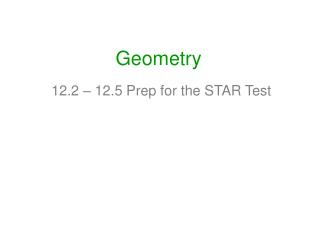Download PresentationGeometry

# Geometry - PowerPoint PPT PresentationDownload Presentation## Geometry

- - - - - - - - - - - - - - - - - - - - - - - - - - - E N D - - - - - - - - - - - - - - - - - - - - - - - - - - -
##### Presentation Transcript

1. Geometry 12.2 – 12.5 Prep for the STAR Test

2. Geometry 12.2 Pyramids

3. 10 6 Let’s solve #7 as we learn the formula for lateral area!! 7) Find the lateral area and total area of this regular pyramid. LA = ½ pl A = ½ b(h) LA = ½ 36(10) A = 3(10) A = 30 LA = 180 square units We have 6 triangles! 10 LA = nF LA = 6(30) 6 LA = 180 square units The lateral area of a regular pyramid with n lateral faces is n times the area of one lateral face. The best way to know the formulas is to understand them rather than memorize them. L.A. = nF OR… The lateral area of a regular pyramid equals half the perimeter of the base times the slant height. Why? Imagine a curtain on the ground around the pyramid(perimeter) and you are pulling the curtain up the slant height. Since the curtain will wrap around triangles we need the ½ . L.A. = ½ pl

4. 10 6 7) Find the lateral area and total area of this regular pyramid. A = ½ a(p) TA = LA + B A = ½ 3√3(36) TA = 180 + 54√3 sq. units A = 3√3(18) 30 3√3 A = 54√3 3 6 The total area of a pyramid is its lateral area plus the area of its base. T.A. = L.A. + B That makes sense!

5. h l Let’s solve #9 as we learn the formula volume!! 9. Find the volume of a regular square pyramid with base edge 24 and lateral edge 24. Draw a square pyramid with the given dimensions. Must be a 30-60-90. V = 1/3 B(h) 1/3 the volume of its prism. V = 1/3 24(24)(h) 122 + x2 = (12√3)2 12√3 12√3 12√2 V = 8(24)(h) 24 V = 192(h) 12 12 V = 192(12√2) 24 V = 2304√2 sq. units The volume of a pyramid equals one third the area of the base times the height of the pyramid. Why? Because a rectangular prism with the same base and same height would be V = bh and the pyramid, believe it or not, would hold 1/3 the amount of water.

6. 12.3 Cylinders and Cones

7. To find lateral area (L.A.): Take a can of soup Peel off the label Multiply circumference by height height circumference

8. To find total area (T.A.): Take the label (LA) Pop the top and find the area of the base Add 2 base areas to the LA A = πr² Top Bottom

9. To find volume (V): Start with the area of the base Multiply it by height That’s how much soup is in the can ! H r A = πr²

10. Lateral Area of a Cylinder: L.A. The lateral area of a cylinder equals the circumference of a base times the height of the cylinder. L.A = 2πrH 8 6 L.A = dπH which is • L.A = CH which is LA = 12π • 8 = 96π square units

11. Total Area of a Cylinder: T.A. The total area of a cylinder is the lateral area plus twice the area of a base. T.A = L.A. + 2B 8 6 • which is TA = 96π + 2(π • 6²) = 96π + 2(36π) = 96π + 72π = 168π square units T.A. = 2πrH + 2πr²

12. Volume of a Cylinder: V The volume of a cylinder equals the area of a base times the height of the cylinder. V = πr²H 8 6 • V = (π • 6²) • 8 = 36π • 8 = 288π cubic units

13. Lateral Area of a Cone: L.A. 10 8 • L.A = πrl 6 LA = π • 6 •10 = 60π square units

14. Total Area of a Cone: T.A. 10 T.A = L.A. + B 8 • 6 which is TA = 60π + (π • 6²) = 60π + 36π = 96π square units T.A. = πrl + πr²

15. Volume of a Cone: V The volume of a cone equals one third the area of the base times the height of the cone. 10 V = πr²h 8 • 6 V = 1/3 • π • 6² • 8 = 96π cubic units

16. 12.4 Spheres

17. Surface Area Formula Surface Area = What does this represent? It represents the area of a circle with radius = r. r

18. Volume Formula Volume = A way to remember which formula is cubed… The volume formula has two 3’s and volume is measured in units3!!

19. 1. radius = 10 Area: _____ Volume: _____ Surface Area = Volume = = 4π(10)2 = 4π(100) = 400πunits2

20. Geometry 12.5 Areas and Volumes of Similar Solids

21. Scale Factor If the scale factor of two solids is a:b, then (1) the ratio of corresponding perimeters is a:b (2) the ratio of base areas, of lateral areas, and of the total area is a²:b² (3) the ratio of volumes is a³:b³ SCALE FACTOR: 1:2 Base circumference: 6π:12π 1:2 Lateral areas: 15π:60π 1:4 Volumes: 12π:96π 1:8 10 8 5 4 • 3 6

22. Exercises Find the missing information. 2:7 5:6 3:10 2:5 2:5 1:9 25:36 9:100 4:25 4:25 1:27 8:343 8:125

23. HW • Midpoint WS